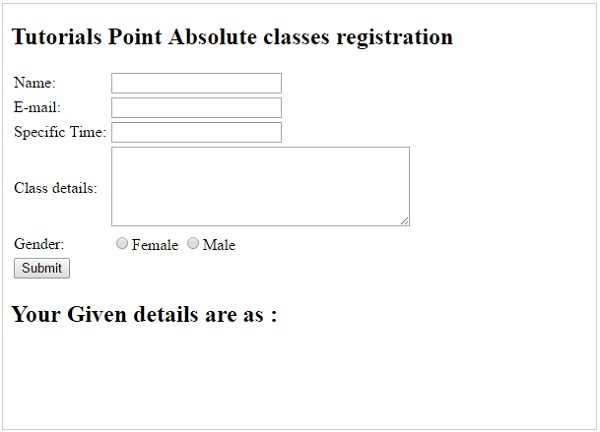# PHP - Form Introduction

## Dynamic Websites

The Websites provide the functionalities that can use to store, update, retrieve, and delete the data in a database.

## What is the Form?

A Document that containing black fields, that the user can fill the data or user can select the data.Casually the data will store in the data base

### Example

Below example shows the form with some specific actions by using post method.

```<html>

<title>PHP Form Validation</title>

<body>
<?php

// define variables and set to empty values
\$name = \$email = \$gender = \$comment = \$website = "";

if (\$_SERVER["REQUEST_METHOD"] == "POST") {
\$name = test_input(\$_POST["name"]);
\$email = test_input(\$_POST["email"]);
\$website = test_input(\$_POST["website"]);
\$comment = test_input(\$_POST["comment"]);
\$gender = test_input(\$_POST["gender"]);
}

function test_input(\$data) {
\$data = trim(\$data);
\$data = stripslashes(\$data);
\$data = htmlspecialchars(\$data);
return \$data;
}
?>

<h2>Tutorials Point Absolute classes registration</h2>

<form method = "post" action = "/php/php_form_introduction.htm">
<table>
<tr>
<td>Name:</td>
<td><input type = "text" name = "name"></td>
</tr>

<tr>
<td>E-mail:</td>
<td><input type = "text" name = "email"></td>
</tr>

<tr>
<td>Specific Time:</td>
<td><input type = "text" name = "website"></td>
</tr>

<tr>
<td>Class details:</td>
<td><textarea name = "comment" rows = "5" cols = "40"></textarea></td>
</tr>

<tr>
<td>Gender:</td>
<td>
<input type = "radio" name = "gender" value = "female">Female
<input type = "radio" name = "gender" value = "male">Male
</td>
</tr>

<tr>
<td>
<input type = "submit" name = "submit" value = "Submit">
</td>
</tr>
</table>
</form>

<?php
echo "<h2>Your Given details are as :</h2>";
echo \$name;
echo "<br>";

echo \$email;
echo "<br>";

echo \$website;
echo "<br>";

echo \$comment;
echo "<br>";

echo \$gender;
?>

</body>
</html>
```

It will produce the following result −